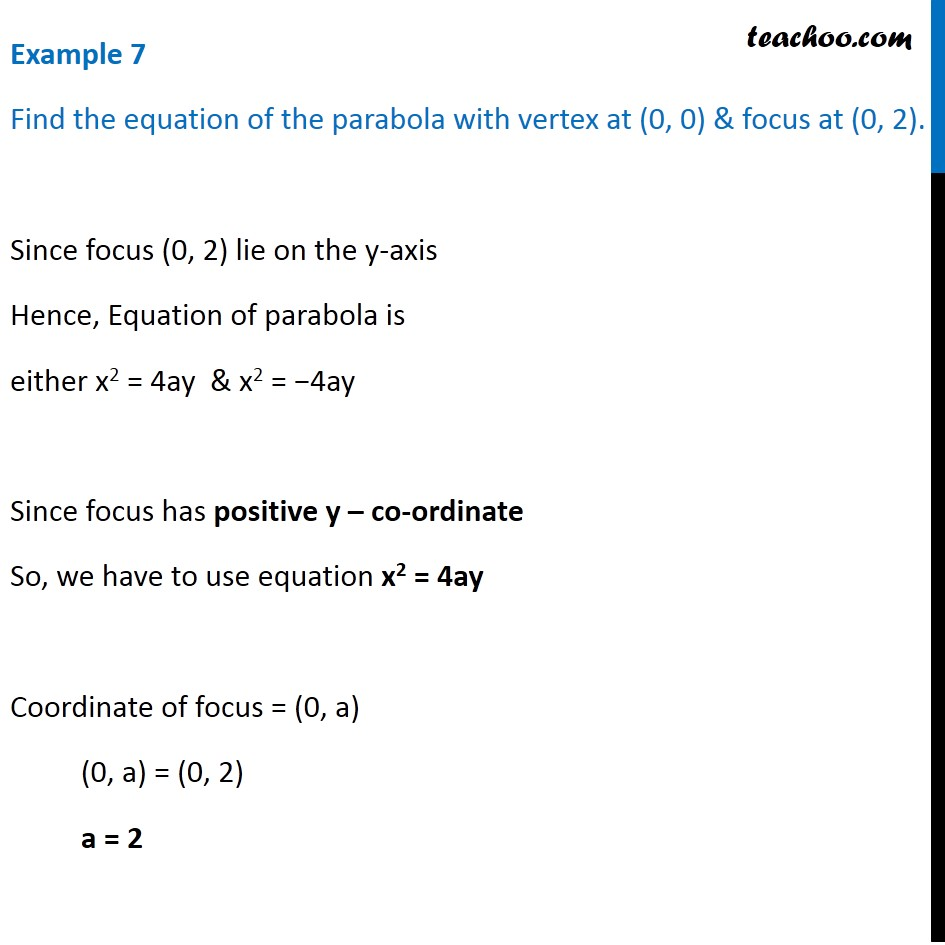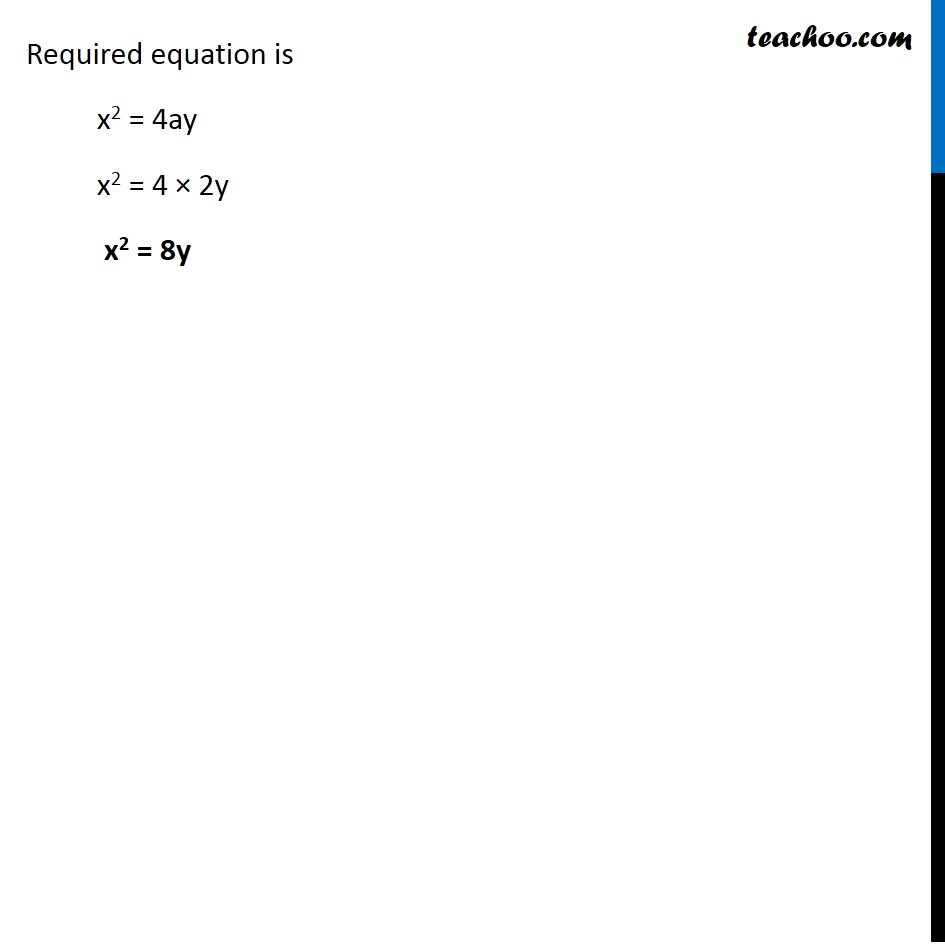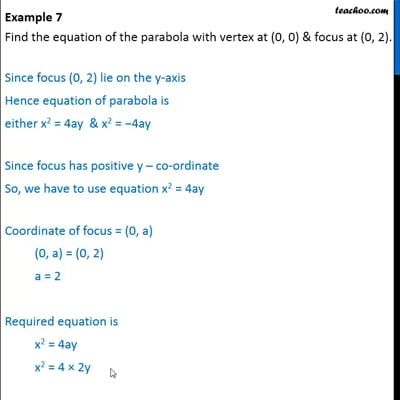Examples

Chapter 11 Class 11 Conic Sections
Serial order wiseThis video is only available for Teachoo black users

Introducing your new favourite teacher - Teachoo Black, at only ₹83 per month

### Transcript

Example 7 Find the equation of the parabola with vertex at (0, 0) & focus at (0, 2). Since focus (0, 2) lie on the y-axis Hence, Equation of parabola is either x2 = 4ay & x2 = −4ay Since focus has positive y – co-ordinate So, we have to use equation x2 = 4ay Coordinate of focus = (0, a) (0, a) = (0, 2) a = 2 Required equation is x2 = 4ay x2 = 4 × 2y x2 = 8y